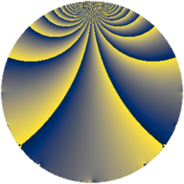# Properties

 Label 69.2.gLevel $69$ Weight $2$ Character orbit 69.g Rep. character $\chi_{69}(5,\cdot)$ Character field $\Q(\zeta_{22})$ Dimension $60$ Newform subspaces $1$ Sturm bound $16$ Trace bound $0$

# Related objects

## Defining parameters

 Level: $$N$$ $$=$$ $$69 = 3 \cdot 23$$ Weight: $$k$$ $$=$$ $$2$$ Character orbit: $$[\chi]$$ $$=$$ 69.g (of order $$22$$ and degree $$10$$) Character conductor: $$\operatorname{cond}(\chi)$$ $$=$$ $$69$$ Character field: $$\Q(\zeta_{22})$$ Newform subspaces: $$1$$ Sturm bound: $$16$$ Trace bound: $$0$$

## Dimensions

The following table gives the dimensions of various subspaces of $$M_{2}(69, [\chi])$$.

Total New Old
Modular forms 100 100 0
Cusp forms 60 60 0
Eisenstein series 40 40 0

## Trace form

 $$60 q - 11 q^{3} - 10 q^{4} - 14 q^{6} - 22 q^{7} - 11 q^{9} + O(q^{10})$$ $$60 q - 11 q^{3} - 10 q^{4} - 14 q^{6} - 22 q^{7} - 11 q^{9} - 22 q^{10} + 4 q^{12} - 22 q^{13} - 46 q^{16} + 12 q^{18} - 22 q^{19} + 22 q^{21} + 50 q^{24} + 8 q^{25} + 10 q^{27} - 22 q^{28} + 33 q^{30} - 22 q^{31} + 22 q^{36} + 22 q^{37} + 13 q^{39} + 132 q^{40} - 11 q^{42} + 22 q^{43} + 66 q^{46} - 58 q^{48} + 68 q^{49} - 11 q^{51} + 94 q^{52} - 33 q^{54} - 44 q^{57} - 8 q^{58} - 121 q^{60} - 66 q^{61} - 66 q^{63} - 20 q^{64} - 66 q^{66} - 44 q^{67} - 66 q^{69} - 132 q^{70} - 101 q^{72} - 44 q^{73} - 44 q^{75} - 110 q^{76} + 84 q^{78} - 66 q^{79} + 77 q^{81} - 132 q^{82} + 77 q^{84} - 44 q^{85} + 73 q^{87} + 66 q^{88} + 176 q^{90} + 116 q^{93} + 100 q^{94} + 85 q^{96} + 44 q^{97} + 121 q^{99} + O(q^{100})$$

## Decomposition of $$S_{2}^{\mathrm{new}}(69, [\chi])$$ into newform subspaces

Label Dim $A$ Field CM Traces $q$-expansion
$a_{2}$ $a_{3}$ $a_{5}$ $a_{7}$
69.2.g.a $60$ $0.551$ None $$0$$ $$-11$$ $$0$$ $$-22$$